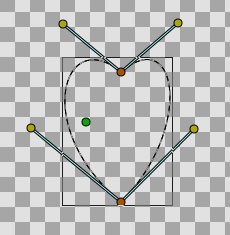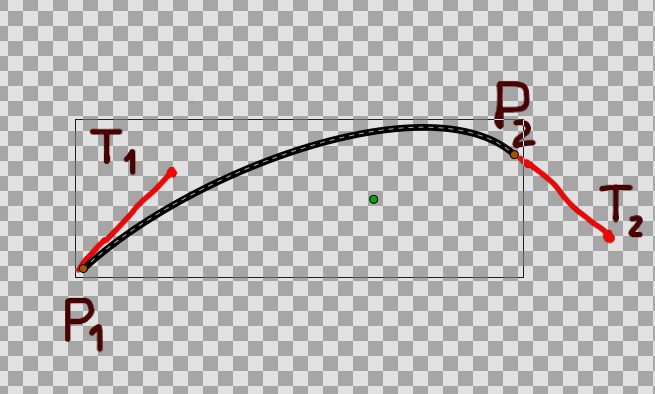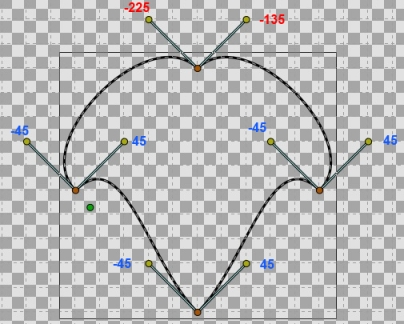# Question on coordinates system

Hi all,

I am not sure where to post this question, this directory seemed the most appropriate.
I am trying to figure out the way curve coordinates are defined but I am a bit puzzled.

I drew the curve shown below and here are the corresponding point parameters :

Point 1
x = 0.5814296007
y =-1.0368576050
T1 radius = 5.0535417411, theta = -42.397438
T2 radius = 4.6251060325, theta = 52.125015

Point 2
x = 0.5408648840
y = 0.6490377784
T1 radius = 2.6889333853, theta = 236.070236
T2 radius = 3.0539710769, theta = 140.389313

What I don’t understand is why the tangent angles are not both referenced with the same origin angle.
What are the rules on tangent angles ?

Thanks

Thierryi never get an eye on that … i’m sure genete / zelgadis could answer you .

maybe you could found some info there ( warning hard math included !)

if you find something interesting , please share your result … we need to document this kind of “vital” informations

1 Like

Thanks for the links, interesting information (beware of the headaches though)

However, these formulas explain how the blines are drawn with existing point and tangent parameters but not how these parameters are set from the user input in the interface. I tried to add 2 more points to my shape and I get tangents referenced to zero “at 12’oclock”. I removed the point which was referenced with zero “at six o’clock” and inserted a new point and here it was again referenced at 6 o’clock. If I put points elsewhere, they are referenced to the 12 o’clock position.

Apparently, there is a segment in the shape where the points are referenced to the 6 o’clock position, everywhere else it is references to 12 o’clock. Could be that the segment is drawn “backwards” compared to the other segments of the shape.
I don’t know if my explanations make sense to anyone else ? If not, it might be caused by all these formulas …!

I’m sorry for the late reply. Lets go to the subject:

Regarding to tangents orientation:
Say you have a spline made with two points P1 and P2 and two tangents T1 and T2. If you draw the spline and its tangents on screen by your own (by hand), you’ll notice that T2 is drawn like that:See how the Spline tool draws each tangent extending the tangent towards the direction of drawing.
But for convenience we draw on screen -T2 because usually tangents on a point are coincident on consecutive splines so if we draw T2 as it is, it would overlap with T1 from the next spline and so we would have problems handling it.

Regarding to angles:
Angles are tricky. And specially in Synfig StudioWhen you manipulate a handle in Synfig the sequence is this: The original value of the handle is cached and only when the user release the mouse (drop the handle again on screen) the new value is stored on the handle. For linear or radial values there is not problem with that, you just replace the old value with the new value and that’s all. But with that simple behavior applied on angles (any angle handle) we would loose the ability of pass 180º value (or just in case 360º). In some handles and parameters from some layers, this is important, because the angle value could be used anywhere and its value beyond 360 can be important (due to Convert types and connection with math formulas). So we decided to include on the code detect the movement of the angles between the four quadrants. If you pass from one quadrant to its consecutive, the trim of the 180 degrees produced by maths (when you calculate the arctang of its x,y position) is not applied and the value is increased consequently.
Say we are on 178 degrees angle and move to 185. With the old algorithm it would result to be -175 and with the new algorithm it is 185.
Said that, angles are coherent with the history of the movements of the tangents by you, the user and different for each situation.

Regarding to the tangent size:
Tangents are drawn by 1/3 of its real length. Is it like that because the real length is excessive long to be manageable. So you have to take account that reduction in order to manually give the values to the spline parameters to draw a particular spline calculated by maths.
(Note to coders: this 1/3 value can be added to the meta data)

I hope it doesn’t hurt too much the brain of the reader.
Cheers.
-G

héhé … i was sure you could easily explain that…Do you think that page wiki.synfig.org/Dev:Spline_Bline is the good one to document this ?

Yes, it is. And may be add a “further reading” link to that page at the end of wiki.synfig.org/Spline_Tool#Othe … nformation
Cheers!
-G

Hi Genete

Thank you for your detailed explanation.

However, there is still one thing I cannot figure : why isn’t the origin of the tangent angles a constant througout a shape.
I added two points to my original heart shape. On the picture, the numbers represent the tangent angle as shown in the properties.Why are the tangent of the upper point different from -45 and 45 degrees ?
Note : on my previous post, I had mentioned the origin being at “12 o’clock”, I had read the angles wrong, the origin is indeed at the usual trigonometric location (my drawing tangents at 45° probably wasn’t the best choice for that matter).

Beyond the reason for the angle being shifted on this one point, is there a way to identify this in the XML file so that I can add the necessary rotation value ?

Thanks !

Can you share the sample file?
-G

Here it is

heart_4_points.sif (7.7 KB)

Hi Genete,

Did the file help figuring out what I meant with the reversed origin ?

Cheers !

Very strange. I can’t figure out why they aren’t similar like the others. :-/
-G

And beyond the reason for this, how does the software figure out which way to interpret the angle values ?

I think I finally figured it out.
Quite simple after all (so many things are obvious once the solution is found !)

It all goes with these rules :

A : Curves “leave” a point along T2 and go “into” a point along T1
B : Origin (zero) angle of T1 is trigonometric PI (“9 o’clock”)
Origin angle of T2 is trigonometric 0 (“3 o’clock”)
Angles are calculated in the trigonometric direction (counter clockwise)

This considered, the values for the shape above become clear.

I am not sure why some angles are expressed in a negative value but it doesn’t really matter. The same angle expressed in a positive value can be obtained by adding 360.

… if you can add that info somewhere in the wiki near spline for example